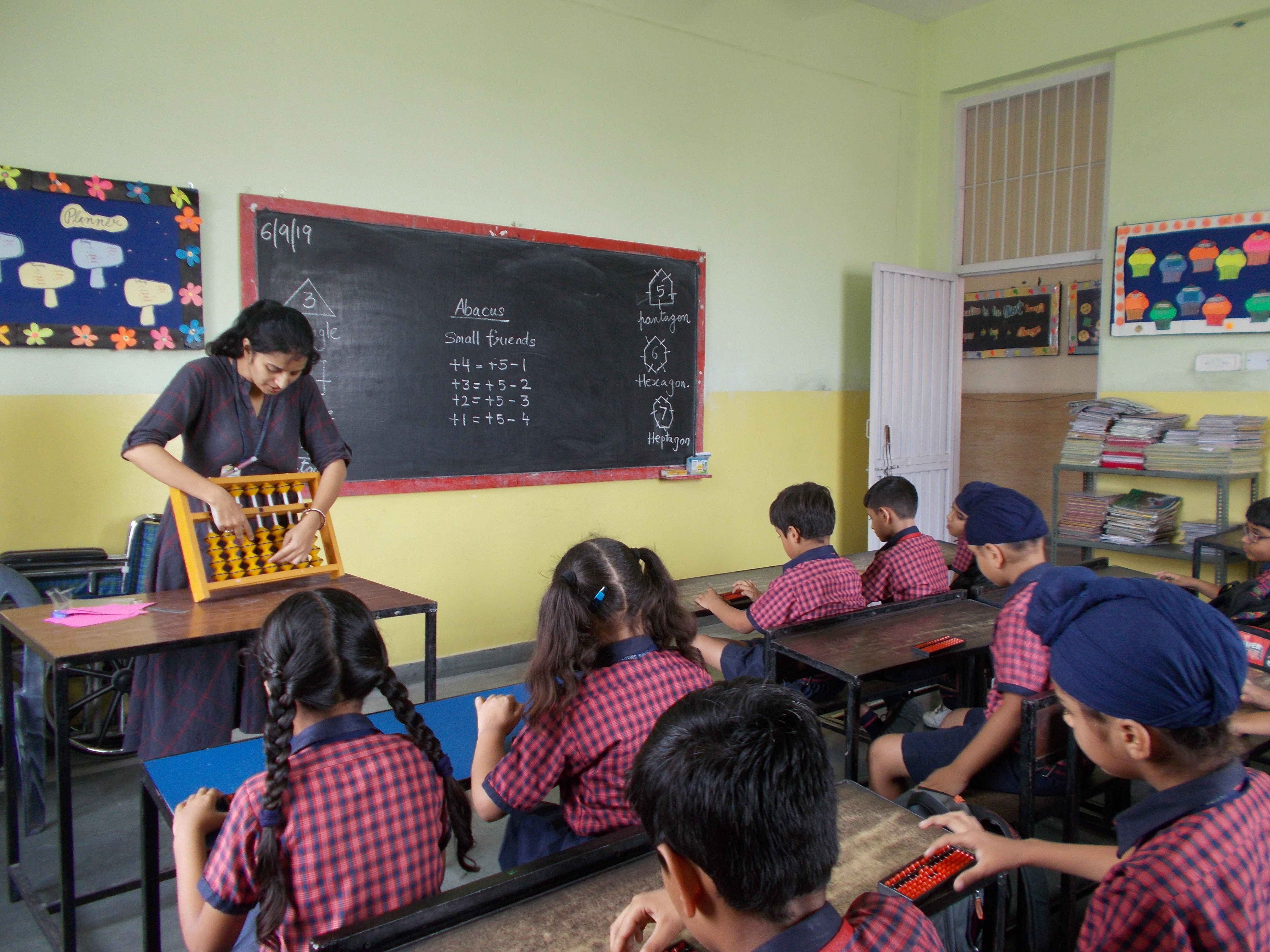•# MATH LAB

•

AKSIPS-125 understands the value of mathaematical operations and its utility in the routine life. So, we emphasize on the practical aspects of learning.The four basic concepts are taught using chinese technique of ABACUS. Various math puzzles are a part of curriculum in primary classes.The mathematics lab provides an opportunity for students to discover mathematics through doing. It is a self explanatory lab with activities in which students takes interest to understand mathematical facts practically through models continue work on the problems/ tasks.

•